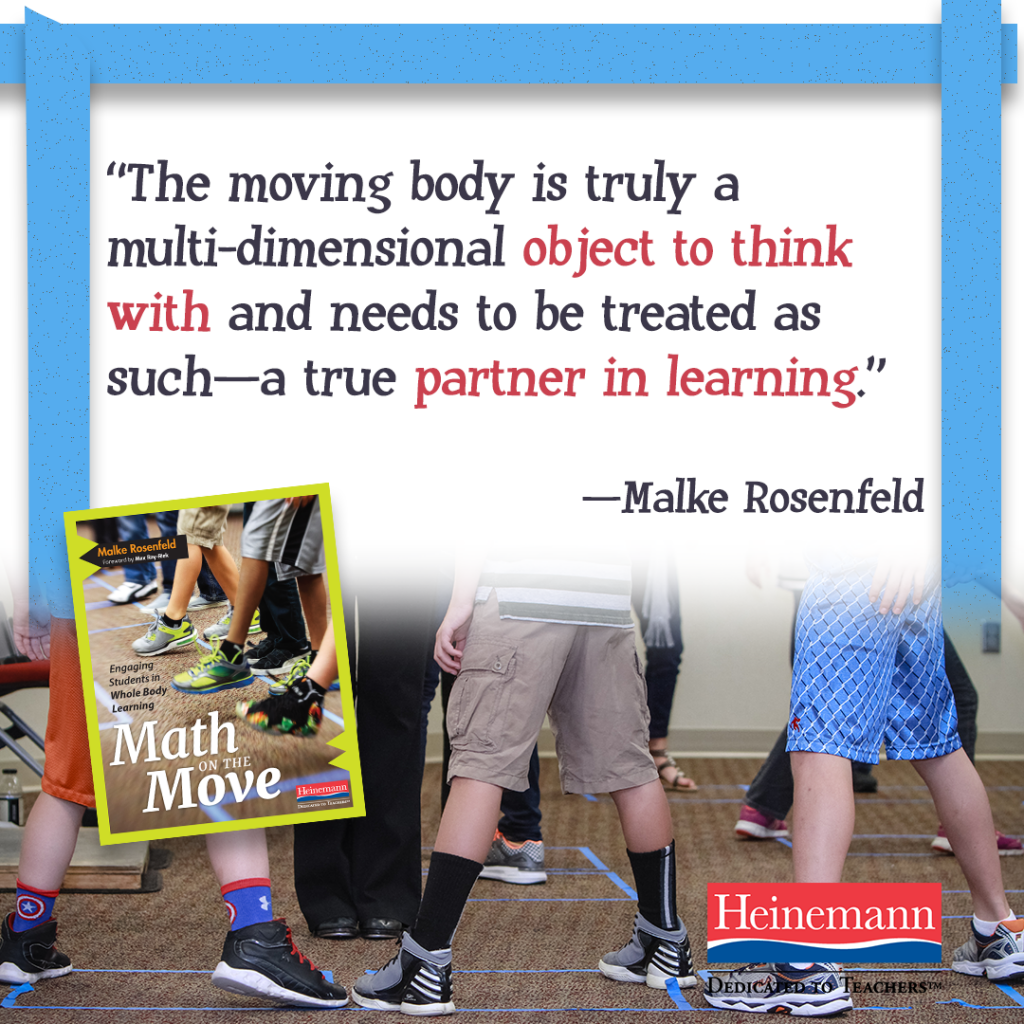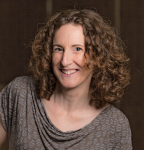<img height="1" width="1" alt="" style="display:none" src="https://www.facebook.com/tr?id=940171109376247&amp;ev=PageView&amp;noscript=1">

# Dedicated to TeachersThe following blog post is adapted from Malke Rosenfeld’s new book, Math on the Move: Engaging Students in Whole Body Learning.

How is this Math?

By Malke Rosenfeld

Because math is frequently presented in a static way in textbooks and on worksheets, the dynamic action and meaning represented by those symbols and figures are often lost in the shuffle. The experience of math as a series of fixed images, ideas, and answers might leave us to wonder: How on earth can we actually learn math if it’s not written down?

When we think of math, what often comes to mind are the nouns, the mathematical objects and procedures that can be named or identified visually. In truth, mathematics is a balance between objects (the nouns) and actions (the verbs). The body is best positioned to express the action side of math, not only in the thinking that happens while doing and learning math, but also in the expression of mathematical concepts that are in and of themselves action oriented. The interwoven relationship between the nouns and verbs of math comes to light when I brainstorm with educators about what kinds of math we might find in dance making. Below is a list of representative educator responses to this type of brainstorming:

 Nouns (objects) Verbs (actions) Patterns (units, cycles) Geometric forms (line, angles, shapes) Fractions (part-whole relationships) Symmetry Direction Spatial reasoning Multiplication (groups) Properties Rate/time Constraint Formation Set/group theory Transform Rotate Reflect

The list on the left always fills up first; the one on the right often has markedly fewer initial entries. I usually have to add words like unitizing, combine, or compare (among others) to the right-hand column after we have identified the relationship between the two lists. Although it might appear as if I’ve set up an either-or situation, the nouns and verbs of math are actually in what Anna Sfard calls a “complementary” relationship, meaning that they are two sides of the same coin (1991, 4). You can’t have one without the other. For example, it is impossible to have a full understanding of symmetry as reproduced in static form on the page.

The action of transformation is an integral part of conceptualizing and generalizing the concept as a whole. Understanding the nature of an angle is another example. Students must synthesize the relationship between three individual but related conceptions: an angle as a static geometric form, as a measure, and as a rotation. The rotation in itself can be hard to conceptualize because that movement is either depicted as a static figure (imagine curved dotted lines between one ray and the other) or enacted by turning an object with no visual clues for the starting or ending position, making it hard to determine the distance or measure of the turn. In a more general sense, mathematics is an activity in which to be engaged as much as subject matter to be learned. The verbs on the action side of math are about the things we do and how we think as we are working mathematically to solve a problem and build a rich conceptual understanding of the discipline at the same time.

You might well be asking yourself, How is this math? or, more to the point perhaps, How is this learning math? In Math on the Move, I try to answer this question by describing and illustrating the kinds of mathematics topics and practices that can be explored, learned, and expressed by the whole, moving body. The categories include the following:

• spatial reasoning and orientation, including experiences of and reasoning about location, distance, direction, and magnitude, all of which support numeracy and mathematical reasoning across topics
• dynamic geometry, including constructions, angles, and rotations
• part-whole relationships (composing and decomposing shapes and numbers)
• equivalence relations, that is, the math of making comparisons while evaluating sameness, similarity, difference, and change, including transformations and symmetry
• mathematical thinking and sense making

Every point on this list can be experienced, explored, and learned in some capacity through the whole, moving body.

♦ ♦ ♦

Want to learn more about Math on the Move and how a moving math classroom creates dynamic new opportunities for learning? Click here to preview a sample chapter.Malke Rosenfeld is a dance teaching artist, author, and presenter whose interests focus on the learning that happens at the intersection of math and the moving body. She delights in creating rich environments in which children and adults can explore, make, play, and talk math based on their own questions and inclinations. Follow her on Twitter @mathinyourfeet.